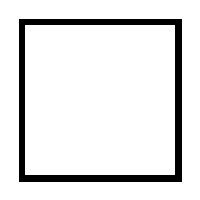Categories

# Induction Squared

I came across an interesting problem recently that I gave to my students in need of enrichment.

Given a square and the ability to divide that square into smaller squares, can you divide a square into ‘n’ smaller squares. The squares do not have to be the same size. For which values of ‘n’ is this possible? For which values of ‘n’ is this impossible?

Students initial reaction was to draw a square and experiment. I cannot think of a better way to begin this problem. It is organic, and contains some very speedy deductions. We begin with suspicion of a pattern, and move into a more regimented induction.Here is our square. There is One of them. The rules state that we can divide any square into smaller squares. The first insight is that only “square” numbers can be created. So each square can be turned into 4,9,16,25, … smaller ones. For example, dividing this square into 4 or 9 would look like this:
It does not take long to discover that 2 and 3 squares are impossible. Four is the closest we can get to 1. Five, Six, are also impossible; When you begin to stumble around in the problem, you find others that are possible. For example, 7 and 12 are possible:
The play reveals some interesting patterns. For example, we begin with 1 square always. Every time we divide it into the smaller squares we lose that original square and create a set of new ones. Therefore, we can only add specific numbers of squares. We can add 3 new squares by dividing an existing square into 4 (4 – the original 1). We can add 8 by splitting into 9. In general, we can add ‘q’ squares to any pattern if:

‘q’ = s -1 , where ‘s’ is a perfect square

This accounts for why 3 was not possible. This also explains why 13 is not possible. The smallest number we can add is 3 (dividing into 4 new squares). We can use this new notation to describe 12 and 7 squares pictured above:

7 = 1 + 3 + 3 (cut original square into 4, then cut another square into 4)
12 = 1 + 8 + 3 (cut original square into 9, then cut another square into 4)

With this framework, we could try chopping forever. Instead, I had to make the realization that if we could represent any 3 consecutive numbers ‘n’, then we could represent every subsequent ‘n’ by simply cutting squares into 4 new squares. This sounds confusing; let me explain.
Because we can always add 3 squares to a formation by cutting one of its squares into 4, we can create 3 roots. Lets say we get a diagram with 14 squares, then 17 is achievable easily by splitting one more into 4–that adds 3 more and 14 + 3 is 17. Twenty is also simple, 23, 26, 29, etc. In fact, every third number would be possible. If we could find three “base cases” in a row we would be able to create any ‘n’ after them by adding successive divisions into 4. With a little playing, I found the first 3 consecutive successes are 15, 16, and 17:
15 = 1+3+3+8 16 = 1+15 17 = 1+8+8

From here, finding a formation is as simple as finding which “base case” it belongs to and cutting enough squares into sets of 4. This provides a proof that any solution above 15 is possible. Trial and error can be used to find those solutions that fit below 15.
Two important things can come from an exercise like this: 1) An appreciation and understanding that we owe mathematical exploration for the underpinning of the result. The process began with trial-and-error and pattern recognition. 2) A collection of further problems can be posed about similar situations:

What about dividing cubes into smaller cubes?
Could we restate this question with other shapes like triangles, rectangles, etc.?
Which numbers have unique representations?
Which ‘n’ has the most representations? Does it have to do with factors?

Mathematics is born from curiosity, suspicion, and stubbornness. All three of these elements are at play with this problem.

NatBanting

## 4 replies on “Induction Squared”

I know this is an old post, but splitting a square into 6 smaller squares is possible, as well as 8 smaller squares. This gives 3 consecutive, 6,7,8. Consider the following, where each x represents one square and the union of o's represent the 6th and 8th squares

x x x
x o o
x o o

x o o o
x o o o
x o o o
x x x x

@boone3936
You can split into 6 squares if you are allowed to erase lines. Maybe I wasn't clear of the rules, but we can only split whole squares into complete grids of smaller squares. So one must be turned into 4,9,16 etc. your method of getting 6 would require a split into 9, then erasing some markings to revert 4 squares into a single square.
If you alter the rules, it makes 6 and 8 possible. In fact, I really like the idea of students challenging the rules and devising a new hypothesis.
Thanks for reading! (Even if it was an earlier post) haha
Nat

I have a question from my son's maths lesson:-

Draw a 5 x 5 square and design a pattern which divides it into nine smaller squares. They cannot overlap and the squares need to be 1×1, 2×2, 3×3 and/or 4×4.

"This also explains why 13 is not possible."

I believe you probably meant to say "14".

13 is simply 1+3+3+3+3, or a cutting into 4 squares 4 times.